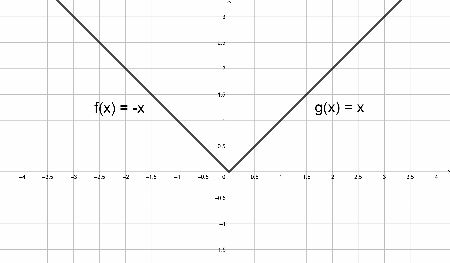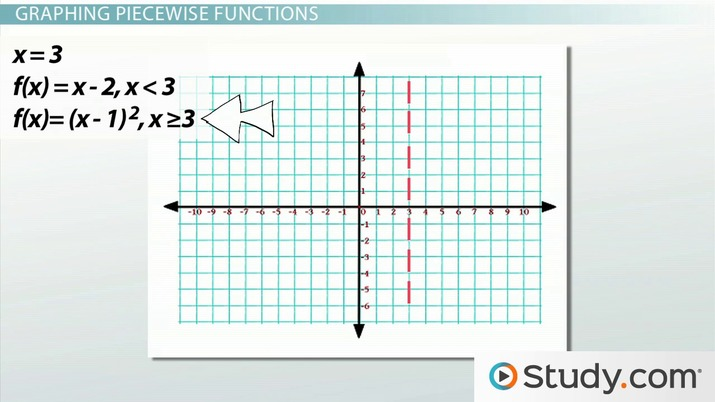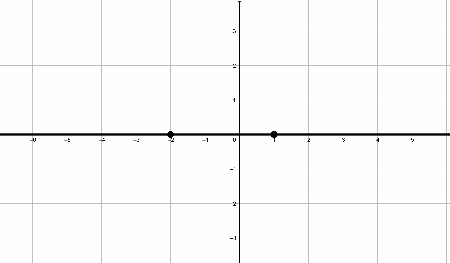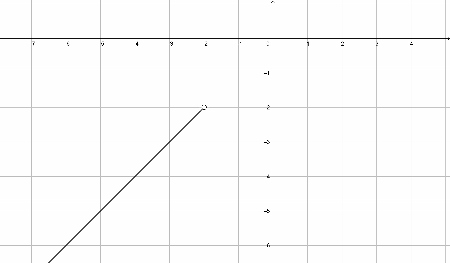# Piecewise Function Graphs

Riley Kench, Jennifer Beddoe
• Author
Riley Kench

Riley has tutored collegiate mathematics for seven years. They have a Master of Arts degree in Mathematics from Central Michigan University and a Bachelor of Science degree in Mathematics from Central Michigan University.

• Instructor
Jennifer Beddoe

Jennifer has an MS in Chemistry and a BS in Biological Sciences.

In this lesson, learn how to graph piecewise functions. Learn to understand the steps involved in graphing piecewise functions and see examples of graphing piecewise functions. Updated: 01/29/2022

Show

## Piecewise Functions

A piecewise function is a function created using two or more functions on distinct domains. That is, a piecewise function is made from two or more functions that are defined on their own domains. Here is what a piecewise function will look like:

• {eq}P(x)= \left\{\begin{matrix} f(x)& D_1 \\ g(x)& D_2 \\ h(x)& D_3 \\ \end{matrix}\right. {/eq}

In the definition above, P is the piecewise function, f, g, and h are each of the pieces (subfunctions) that make up the piecewise function, and the D's represent the distinct domains on which the pieces are defined (subdomains). The subdomains cannot overlap but oftentimes the domain of one subfunction will end where another one begins. If the subdomains did overlap, then the piecewise function would no longer be a function.

Normal functions are singular equations with one domain, while piecewise functions contain several different functions all defined on different domains.

Here is an example illustrating piecewise functions:

Example:

{eq}P(x)= \left\{\begin{matrix} -x ;& x < 0 \\ x ;& x \geq 0 \\ \end{matrix}\right. {/eq}

This piecewise function has two pieces: {eq}f(x) = -x {/eq} and {eq}g(x) = x {/eq}. Each of these pieces have unique domains that do not overlap. Figure 1, below, shows this piecewise function:Notice that the graph in Figure 1 is also the graph of the absolute value function. The absolute value function can therefore also be defined as a piecewise function. The two pieces in the image are also labeled and they are only defined on their domains. The two pieces meet at the origin since the two pieces share the same output at that point.An error occurred trying to load this video.

Try refreshing the page, or contact customer support.

Coming up next: Translating Piecewise Functions

### You're on a roll. Keep up the good work!

Replay
Your next lesson will play in 10 seconds
• 0:06 What Is a Function?
• 1:08 What Is a Piecewise Function?
• 1:50 Graphing Piecewise Functions
• 3:29 Three Is Not a Crowd
• 4:31 Lesson Summary
Save Save

Want to watch this again later?

Timeline
Autoplay
Autoplay
Speed Speed

## How to Graph Piecewise Functions

In this section, we will look at how to graph piecewise functions step by step. Firstly, the graph of a piecewise function is comprised of distinct pieces defined on different domains, rather than one piece that arises with regular functions. Where a regular function has one singular domain, the piecewise function has multiple subdomains that define each piece. They are typically written after each function piece in the piecewise function equation. The process for graphing a piecewise function is similar to that of graphing regular functions.

Here is a step by step procedure for graphing piecewise functions:

• First, identify the given domains and intervals on which each piece of the piecewise function is defined. These will be of the form {eq}(a,b) {/eq}, {eq}(a,b] {/eq}, {eq}[a,b) {/eq}, or {eq}[a,b] {/eq}. It may be helpful to show on the x-axis the intervals when graphing a piecewise function.
• Next, determine which functions apply to each interval and domain found in the previous step. Then, use the functions to find inputs and outputs for the indicated intervals.
• Finally, graph each function piece on its corresponding interval. Once all function pieces have been graphed on one grid, the piecewise graph is finished. The final image is a piecewise graph.

This process for how to graph piecewise functions essentially extends the process of graphing one function defined on the real numbers to multiple functions defined on subsets of the real line. Oftentimes, the functions are continuous and only need to be restricted to certain intervals of the real line.

The next section will explore examples of how to graph piecewise functions.

## Graphing Piecewise Functions Examples

The following are examples of how to graph piecewise functions, with accompanying graphs showing the steps involved:

Example 1:

Consider the piecewise function: {eq}P(x)= \left\{\begin{matrix} x; & x<-2 \\ 3-x^2; & -2 \leq x \leq 1 \\ 1; & x > 1 \\ \end{matrix}\right. {/eq}. What would be the graph of this piecewise function?

Solution:

• The first step is to identify the intervals for each subdomain. They are: {eq}(-\infty, -2) {/eq}, {eq}[-2,1] {/eq}, and {eq}(1, \infty) {/eq}. These can be found after each function. Simply translate the inequalities into interval notation. Figure 2 shows these intervals on one grid:• In the image in Figure 2, the intervals are shown on the x-axis with points marked to show the endpoints of each interval. If the line goes off towards infinity, the interval also goes off towards infinity.
• The next step is to associate to each interval a subfunction from the piecewise function: {eq}x {/eq} is associated to the interval {eq}(-\infty, -2) {/eq}, {eq}3-x^2 {/eq} is associated to the interval {eq}[-2, 1] {/eq}, and {eq}1 {/eq} is associated to the interval {eq}(1, \infty) {/eq}.
• Now, graph each piece of the piecewise function in order to build the piecewise graph. Start with the first subfunction of {eq}x {/eq}. This has a slope of one and crosses the point {eq}(-3,-3) {/eq} but does not pass through {eq}(-2,-2) {/eq} since this point is not part of the interval in question. Figure 3 shows this piece of the piecewise function graphed:• The graph in Figure 3 shows the graph of {eq}y=x {/eq} restricted to the interval {eq}(-\infty, -2) {/eq}.

To unlock this lesson you must be a Study.com Member.

#### What is a piecewise function example?

For example, consider the piecewise function defined by p(x) = { -x if x< 0 and x if x is greater than or equal to zero. The two intervals in this example are (-infinity, 0) and 0, infinity). Graphing y=-x first on the interval (-infinity, 0) will yield the first part of the graph. Then, graph y=x on the interval [0,infinity) to get the last piece. This will also be the graph of the absolute value function; this is another way to define it. Once both pieces have been graphed, the piecewise graph is done.

#### How do you graph piecewise functions?

To graph a piecewise function, first identify the domains of each subfunction using intervals. Then, use each function and each of their corresponding intervals to graph each piece of the piecewise function. Any points omitted by the interval are not graphed by the function, as they will typically be included in other intervals.

#### How do you graph two piecewise functions?

Firstly, identify each of the domains corresponding to the different subfunctions of the piecewise function. Then, look at the inputs and outputs of each of the functions and their corresponding intervals. Then, graph each piece of the piecewise function defined on each interval. Once all pieces have been graphed, the final piecewise graph is done.

### Register to view this lesson

Are you a student or a teacher?